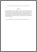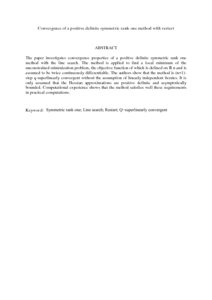Simple Search:

Convergence of a positive definite symmetric rank one method with restart

Citation

Leong, Wah June and Abu Hassan, Malik (2009) Convergence of a positive definite symmetric rank one method with restart. Advance Modeling and Optimization, 11 (4). pp. 423-433. ISSN 1841-4311

Abstract / Synopsis

The paper investigates convergence properties of a positive definite symmetric rank one method with the line search. The method is applied to find a local minimum of the unconstrained minimization problem, the objective function of which is defined on ℝ n and is assumed to be twice continuously differentiable. The authors show that the method is (n+1)-step q-superlinearly convergent without the assumption of linearly independent iterates. It is only assumed that the Hessian approximations are positive definite and asymptotically bounded. Computational experience shows that the method satisfies well these requirements in practical computations.Preview
PDF (Abstract)
Convergence of a positive definite symmetric rank one method with restart.pdfView Item